# CAT Questions | CAT Geometry Questions

###### CAT Quantitative Aptitude | CAT Geometry Triangles , Circles

A CAT Geometry question that appears in the Quantitative Aptitude section of the CAT Exam broadly tests an aspirant on the concepts - Triangles, Circles, Quadrliaterals, Polygons & mixture of the above mentioned concepts. In CAT Exam, one can generally expect to get 4~6 questions from CAT Geometry. CAT Geometry is an important topic with lots of weightage in the CAT Exam. Make use of 2IIMs Free CAT Questions, provided with detailed solutions and Video explanations to obtain a wonderful CAT score. If you would like to take these questions as a Quiz, head on here to take these questions in a test format, absolutely free.

1. #### CAT Geometry: Obtuse Triangles - Integers

x, y, z are integer that are side of an obtuse-angled triangle. If xy = 4, find z.

1. 2
2. 3
3. 1
4. More than one possible value of z exists

2. #### CAT Geometry: Isosceles Triangles - Integers

How many isosceles triangles with integer sides are possible such that sum of two of the side is 12?

1. 11
2. 6
3. 17
4. 23

3. #### CAT Geometry: Triangles - Area

Sides of a triangle are 6, 10 and x for what value of x is the area of the △ the maximum?

1. 8 cms
2. 9 cms
3. 12 cms
4. None of these

4. #### CAT Geometry: Equilateral triangle and circle

Two circles are placed in an equilateral triangle as shown in the figure. What is the ratio of the area of the smaller circle to that of the equilateral triangle

1. π:36√3
2. π:18√3
3. π:27√3
4. π:42√3

5. #### CAT Geometry: Triangles - Perimeter

Perimeter of a △ with integer sides is equal to 15. How many such triangles are possible?

1. 7
2. 6
3. 8
4. 5

6. #### CAT Geometry: Equilateral Triangle and Square

There is an equilateral triangle with a square inscribed inside it. One of the sides of the square lies on a side of the equilateral △. What is the ratio of the area of the square to that of the equilateral triangle?

1. 12 : 12 + 7√3
2. 24 : 24 + 7√3
3. 18 : 12 + 15√3
4. 6 : 6 + 5√3

7. #### CAT Geometry: Triangles - Integers

△ABC has integer sides x, y, z such that xz = 12. How many such triangles are possible?

1. 8
2. 6
3. 9
4. 12

8. #### CAT Geometry: Regular Polygon

ABCDE is a regular pentagon. O is a point inside the pentagon such that AOB is an equilateral triangle. What is ∠OEA?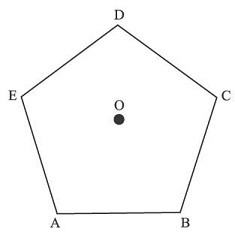1. 66°
2. 48°
3. 54°
4. 72°

9. #### CAT Geometry: Triangles Properties

△ has sides a2, b2 and c2. Then the triangle with sides a, b, c has to be:

1. Right-angled
2. Acute-angled
3. Obtuse-angled
4. Can be any of these three

10. #### CAT Geometry: Right Triangle Properties

Consider a right–angled triangle with inradius 2 cm and circumradius of 7 cm. What is the area of the triangle?

1. 32 sq cms
2. 31.5 sq cms
3. 32.5 sq cms
4. 33 sq cms

11. #### CAT Geometry: Regular Polygon

What is the ratio of longest diagonal to the shortest diagonal in a regular octagon?

1. √3 : 1
2. 2 : 1
3. 2 : √3
4. √2 : 1

12. #### CAT Geometry: Altitude of a Triangle

Find the altitude to side AC of triangle with side AB = 20 cm, AC = 20 cm, BC = 30 cm.

1. 10√7
2. 8√7
3. 7.5√7
4. 15√7

13. #### CAT Geometry: Regular Polygon

ABCDEF is a regular hexagon inscribed inside a circle. If the shortest diagonal of the hexagon is of length 3 units, what is the area of the shaded region.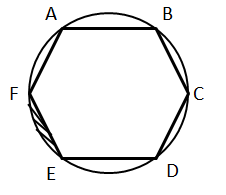1. 1/6(3π − (9√3)/2)
2. 1/6(2π − (6√3)/2)
3. 1/6(3π − (8√3)/2)
4. 1/6(6π − (15√3)/2)

14. #### CAT Geometry: Circles

A circle of radius 5 cm has chord RS at a distance of 3 units from it. Chord PQ intersects with chord RS at T such that TS = 1/3 of RT. Find minimum value of PQ.

1. 6√3
2. 4√3
3. 8√3
4. 2√3

15. #### CAT Geometry: Triangles - Area

Triangle has perimeter of 6 + 2√3 . One of the angles in the triangle is equal to the exterior angle of a regular hexagon another angle is equal to the exterior angle of a regular 12-sided polygon. Find area of the triangle.

1. 2√3
2. √3
3. √3/2
4. 3

Area of a Rhombus of perimeter 56 cms is 100 sq cms. Find the sum of the lengths of its diagonals

1. 33.40
2. 34.40
3. 31.20
4. 32.30

Rhombus has a perimeter of 12 and one angle = 120°. Find its area.

1. 9 * (√3)/2
2. 3 * (√3)/2
3. 9 * √3
4. 18 * √3

18. #### CAT Geometry: Triangles - Area

Circle with center O and radius 25 cms has a chord AB of length of 14 cms in it. Find the area of triangle AOB

1. 144 cm2
2. 121 cm2
3. 156 cm2
4. 168 cm2

19. #### CAT Geometry: Circles

Two mutually perpendicular chords AB and CD intersect at P. AP = 4, PB = 6, CP = 3. Find radius of the circle.

1. 31.25(1/2)
2. 37.5(1/2)
3. 26(1/2)
4. 52(1/2)

20. #### CAT Geometry: Triangles

Triangle ABC has angles A = 60° and B = 70°. The incenter of this triangle is at I. Find angle BIC.

1. 90°
2. 130°
3. 80°
4. 120°

Rhombus of side 6 cm has an angle equal to the external angle of a regular octagon. Find the area of the rhombus.

1. 18√2 cm2
2. 9√2 cm2
3. 15√2 cm2
4. 12√2 cm2

22. #### CAT Geometry: Circle, Square and Triangle

A circle inscribed in a square of side 2 has an equilateral triangle inscribed inside it. What is the ratio of areas of the equilateral triangle to that of the square?

1. 9√3 : 16
2. 3√3 : 4
3. 9√3 : 4
4. 3√3 : 16

23. #### CAT Geometry: Isosceles Triangles

An acute-angled isosceles triangle has two of its sides equal to 10 and 16. Find the area of this triangle.

1. √231 units
2. 12√66 units
3. 24 units
4. 5√231 units

24. #### CAT Geometry: Triangles - Area

Three equal circles are placed inside an equilateral triangle such that any circle is tangential to two sides of the equilateral triangle and to two other circles. What is the ratio of the areas of one circle to that of the triangle?

1. π : (6+4√3)
2. 3π : (6+4√3)
3. 2π : (6+4√3)
4. π : (6+2√3)

25. #### CAT Geometry: Triangle and Square

There is an equilateral triangle with a square inscribed inside it. One of the sides of the square lies on a side of the equilateral △. What is the ratio of the area of the square to that of the equilateral triangle?

1. √3 : (5 + 4√3)
2. 2√3 : (7 + 4√3)
3. 4√3 : (7 + 4√3)
4. 4√3 : (5 + 2√3)

26. #### CAT Geometry: Squares and Circles

Consider Square S inscribed in circle C, what is the ratio of the areas of S and Q? And, Consider Circle C inscribed in Square S, what is the ratio of the areas of S and Q?

1. 2:π, 4:π
2. 4:π, 2:π
3. 1:π, 4:π
4. 2:π, 1:π

27. #### CAT Geometry: Triangles - Area

Consider equilateral triangle T inscribed in circle C, what is ratio of the areas of T and C? Consider Circle C inscribed in equilateral triangle T, what is ratio of the areas of T and C?

1. 3√3:π , 3√3:16π
2. 3√3:4π , 3√3:π
3. √3:π , 3√3:4π
4. √3:π , √3:16π

28. #### CAT Geometry: Regular Octagon

Consider Regular Hexagon H inscribed in circle C, what is ratio of the areas of H and C? Consider Circle C inscribed in Regular Hexagon H, what is ratio of the areas of H and C?

1. 2√3 : 3π , 3√3 : 4π
2. 3√3 : π , 3√3 : 4π
3. 3√3 : 2π, 2√3 : π
4. √3 : π , √3 : 4π

29. #### CAT Geometry: Triangles

What is the distance between the orthocentre and the circumcenter of a triangle who sides measure 24 cm, 26 cm and 10 cm?

1. 13 cm
2. 12 cm
3. 7.5 cm
4. √30 cm

30. #### CAT Geometry: Circles and Triangles

Two circles with centres O1 and O2 touch each other externally at a point R. AB is a tangent to both the circles passing through R. P’Q’ is another tangent to the circles touching them at P and Q respectively and also cutting AB at S. PQ measures 6 cm and the point S is at distance of 5 cms and 4 cms from the centres of the circles. What is the area of the triangle SO1O2?

1. 9 cm2
2. 3(4+√7)/2 cm2
3. 27/2 cm2
4. (3√41)/2 cm2

31. #### CAT Geometry: Circles

What is the circumference of the below circle given that AB is the diameter and XY is perpendicular to AB?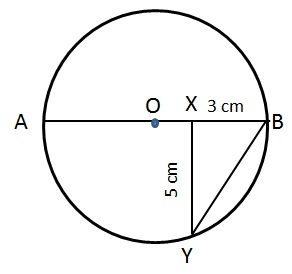1. 8π cm
2. π√34 cm
3. 34π/3 cm
4. π√31/3 cm

32. #### CAT Geometry: Circles

Find ∠PRB. Given
I. ∠BPQ = 22 and O is the centre of the circle
II. ∠RBP = 54 and chord PQ is parallel to AB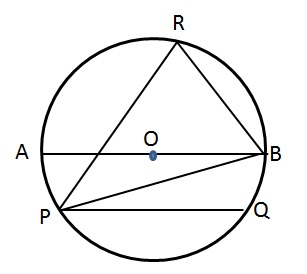1. Either I or II individually is sufficient
2. Both I and II together are required
3. One of the statements alone is sufficient
4. Need more data

33. #### CAT Geometry: Triangles

The two sides of a triangle are 8 cm and 9 cm and one angle is 60. Which of the following can be the length of its third side?
I. √23 cm
II. √73 cm
III.(4.5 - √3.25) cm
IV. (4 + √33) cm
V. (9 + √13) cm

1. Only II and IV
2. Only I and III
3. Only I, II and V
4. Only II, III and IV

There is a set of parallel lines with x lines in it and another set of parallel lines with y lines in it. The lines intersect at 12 points. If x > y, find the maximum number of parallelograms that can be formed.

1. 16
2. 15
3. 18
4. 33

35. #### CAT Geometry: Polygon and Circles

There are 2 concentric circles, one big and one small. A square ABCD is inscribed inside the big circle while the same square circumscribes the small circle. The square touches the small circle at points P, Q, R and S. Determine the ratio of circumference of big circle to the polygon PQRS.

1. π : 2
2. 2 : π
3. 2 : √2
4. π : √2

If PQ || RS, find the value of x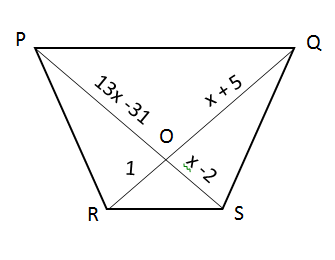1. 7
2. 3
3. Both A & B
4. None of these

37. #### CAT Geometry: Circles

A circle is inscribed in a semi-circle as shown:-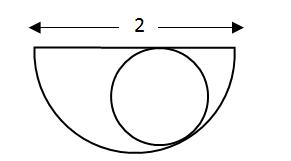The radius of the circle is:-

1. (√2 + 1)/2
2. √2 − 1/2
3. 1 − √2
4. √2 − 1

38. #### CAT Geometry: Circles

Two circles of radius 5 cm have a direct tangent PQ and an indirect tangent RS. Find the length of PQ if RS = 24 cm.

1. 29 cm
2. 13 cm
3. 26 cm
4. Cannot be determined due to lack of information

39. #### CAT Geometry: Circles and Triangles

In the below figure which of the following holds good?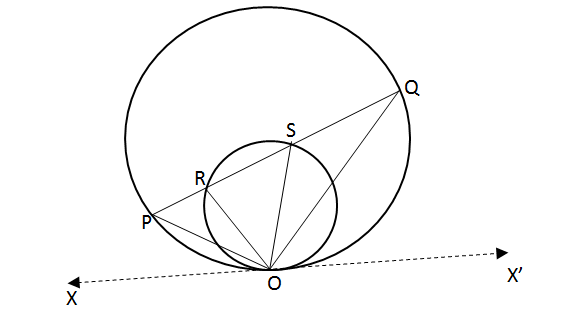1. ∠SQO = ∠ROP
2. 2 ∠ROP = ∠SOR
3. ∠POR = ∠ASO
4. ∠QOX’ = ∠SOR + ∠ROP

In the below figure, If $$frac{PQ}{PR}\\$ = $\frac{PR}{RQ}\\$, then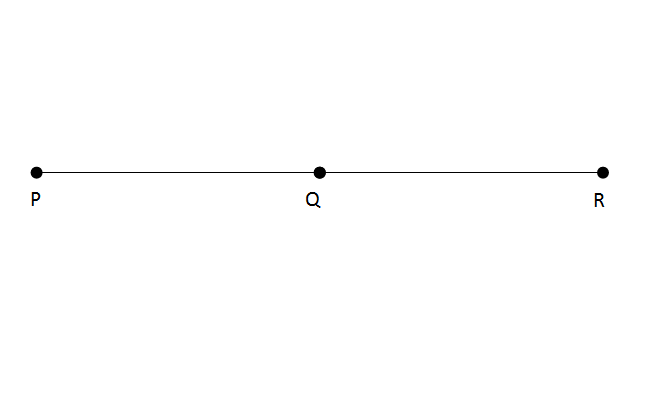1. $\frac{PR}{QR}\\$ > 2 2. $\frac{PR}{QR}\\$ = 2 3. $\frac{PR}{QR}\\$ < 2 4. can’t be determined 41. #### CAT Geometry: Triangles A right angled triangle PQR is such that ∠PRQ = 90° and QR = 4 cm T is a point on QR such that PT = 3 cm, and perimeter of triangle PQT = Perimeter of triangle PTR Then, QT/TR takes the value: 1. QT/TR < 1/3 2. 1/3 < QT/TR < 1 3. QT/TR > 1 4. can’t be determined 42. #### CAT Geometry: Triangles M and N are two points on the side PQ and PR of a triangle PQR respectively such that MNQR is a trapezium and MN:QR = 2:5. Find the ratio of the area of triangle PMN : Trapezium MNQR. 1. 4:25 2. 1:2 3. 4:21 4. 4:10 43. #### CAT Geometry: Triangles In the below figure, ΔABC is right angled and AC = 100 cm. Also, AD = DE = EF = FC. Find the value of: BD2 + BE2 + BF2$in cm2)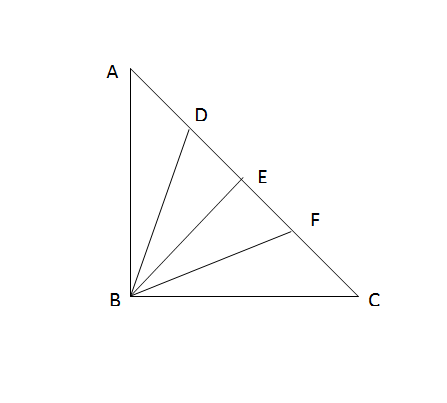1. 10,000 cm2
2. 5,000 cm2
3. 8,750 cm2
4. 12,500 cm2

44. #### CAT Geometry: Triangles

The Olympics committee came up with a new rule. The flag of the gold medal winning team would be hoisted to the right (AB) at 5m. The flag of silver medal winning team would be hoisted to the left (PQ) at a height of 3m. The flag (MN) of bronze medal winning team would be hoisted at the point of intersection of the line joining the top of each of AB and PQ to the foot of other, as shown in the figure below. A and P are 8m apart. In a wrestling event, India won the bronze medal. Find the height at which the Indian flag was hoisted.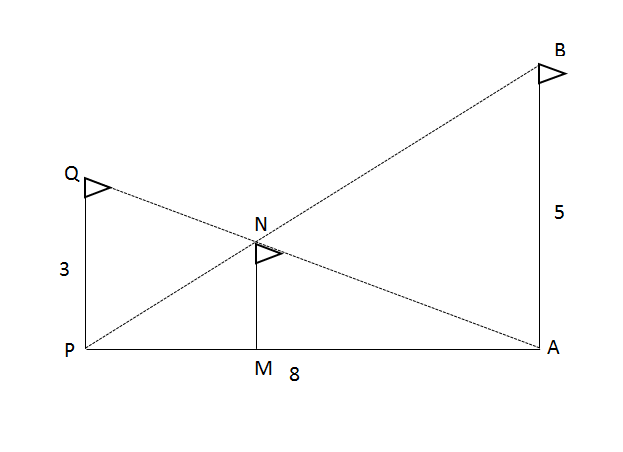1. 2 m
2. $$frac{5}{2}\\$ m 3. $\frac{5}{8}\\$ m 4. $\frac{15}{8}\\$ m 45. #### CAT Geometry: Polygons The number of sides in a regular polygon is ‘T’ times the number of diagonals in it. What is the interior angle of this polygon in terms of T? 1. 180 * $\frac{$T+2$}{(3T+2)}$ 2. 540 * $\frac{$T+2$}{(3T+2)}$ 3. 360 * $\frac{$T+2$}{(3T+2)}$ 4. 90 * $\frac{$T+2$}{(3T+2)}$ ###### 2IIM's Online CAT Coaching4000 off on CAT Online Course for CAT 2020. Vaild till Dec 16th Signup Now! ###### Already have an Account? ###### CAT Coaching in ChennaiCAT 2020Enroll at 44,000/- Next Weekend Batch Starts Sat, Dec 14th, 2019 ###### Best CAT Coaching in ChennaiRegister Online, get Rs 4000/- off Attend a Demo Class ## CAT Preparation Online | CAT Geometry questions Videos On YouTube #### Other useful sources for Geometry Question | Geometry Triangles Circles Quadrilaterals Sample Questions ##### Where is 2IIM located? 2IIM Online CAT Coaching A Fermat Education Initiative, 58/16, Indira Gandhi Street, Kaveri Rangan Nagar, Saligramam, Chennai 600 093 ##### How to reach 2IIM? Phone:$91) 44 4505 8484
Mobile: (91) 99626 48484
WhatsApp: WhatsApp Now
Email: prep@2iim.com

CAT Questions - Geometry Questions: Triangles, 2IIM CAT 2019 online classes, CAT coaching in Chennai | 2IIM Online CAT Coaching

# CAT Questions | CAT Geometry Questions

###### CAT Quantitative Aptitude | CAT Geometry Triangles , Circles

A CAT Geometry question that appears in the Quantitative Aptitude section of the CAT Exam broadly tests an aspirant on the concepts - Triangles, Circles, Quadrliaterals, Polygons & mixture of the above mentioned concepts. In CAT Exam, one can generally expect to get 4~6 questions from CAT Geometry. CAT Geometry is an important topic with lots of weightage in the CAT Exam. Make use of 2IIMs Free CAT Questions, provided with detailed solutions and Video explanations to obtain a wonderful CAT score. If you would like to take these questions as a Quiz, head on here to take these questions in a test format, absolutely free.

1. #### CAT Geometry: Obtuse Triangles - Integers

x, y, z are integer that are side of an obtuse-angled triangle. If xy = 4, find z.

1. 2
2. 3
3. 1
4. More than one possible value of z exists

2. #### CAT Geometry: Isosceles Triangles - Integers

How many isosceles triangles with integer sides are possible such that sum of two of the side is 12?

1. 11
2. 6
3. 17
4. 23

3. #### CAT Geometry: Triangles - Area

Sides of a triangle are 6, 10 and x for what value of x is the area of the △ the maximum?

1. 8 cms
2. 9 cms
3. 12 cms
4. None of these

4. #### CAT Geometry: Equilateral triangle and circle

Two circles are placed in an equilateral triangle as shown in the figure. What is the ratio of the area of the smaller circle to that of the equilateral triangle

1. π:36√3
2. π:18√3
3. π:27√3
4. π:42√3

5. #### CAT Geometry: Triangles - Perimeter

Perimeter of a △ with integer sides is equal to 15. How many such triangles are possible?

1. 7
2. 6
3. 8
4. 5

6. #### CAT Geometry: Equilateral Triangle and Square

There is an equilateral triangle with a square inscribed inside it. One of the sides of the square lies on a side of the equilateral △. What is the ratio of the area of the square to that of the equilateral triangle?

1. 12 : 12 + 7√3
2. 24 : 24 + 7√3
3. 18 : 12 + 15√3
4. 6 : 6 + 5√3

7. #### CAT Geometry: Triangles - Integers

△ABC has integer sides x, y, z such that xz = 12. How many such triangles are possible?

1. 8
2. 6
3. 9
4. 12

8. #### CAT Geometry: Regular Polygon

ABCDE is a regular pentagon. O is a point inside the pentagon such that AOB is an equilateral triangle. What is ∠OEA?1. 66°
2. 48°
3. 54°
4. 72°

9. #### CAT Geometry: Triangles Properties

△ has sides a2, b2 and c2. Then the triangle with sides a, b, c has to be:

1. Right-angled
2. Acute-angled
3. Obtuse-angled
4. Can be any of these three

10. #### CAT Geometry: Right Triangle Properties

Consider a right–angled triangle with inradius 2 cm and circumradius of 7 cm. What is the area of the triangle?

1. 32 sq cms
2. 31.5 sq cms
3. 32.5 sq cms
4. 33 sq cms

11. #### CAT Geometry: Regular Polygon

What is the ratio of longest diagonal to the shortest diagonal in a regular octagon?

1. √3 : 1
2. 2 : 1
3. 2 : √3
4. √2 : 1

12. #### CAT Geometry: Altitude of a Triangle

Find the altitude to side AC of triangle with side AB = 20 cm, AC = 20 cm, BC = 30 cm.

1. 10√7
2. 8√7
3. 7.5√7
4. 15√7

13. #### CAT Geometry: Regular Polygon

ABCDEF is a regular hexagon inscribed inside a circle. If the shortest diagonal of the hexagon is of length 3 units, what is the area of the shaded region.1. 1/6(3π − (9√3)/2)
2. 1/6(2π − (6√3)/2)
3. 1/6(3π − (8√3)/2)
4. 1/6(6π − (15√3)/2)

14. #### CAT Geometry: Circles

A circle of radius 5 cm has chord RS at a distance of 3 units from it. Chord PQ intersects with chord RS at T such that TS = 1/3 of RT. Find minimum value of PQ.

1. 6√3
2. 4√3
3. 8√3
4. 2√3

15. #### CAT Geometry: Triangles - Area

Triangle has perimeter of 6 + 2√3 . One of the angles in the triangle is equal to the exterior angle of a regular hexagon another angle is equal to the exterior angle of a regular 12-sided polygon. Find area of the triangle.

1. 2√3
2. √3
3. √3/2
4. 3

Area of a Rhombus of perimeter 56 cms is 100 sq cms. Find the sum of the lengths of its diagonals

1. 33.40
2. 34.40
3. 31.20
4. 32.30

Rhombus has a perimeter of 12 and one angle = 120°. Find its area.

1. 9 * (√3)/2
2. 3 * (√3)/2
3. 9 * √3
4. 18 * √3

18. #### CAT Geometry: Triangles - Area

Circle with center O and radius 25 cms has a chord AB of length of 14 cms in it. Find the area of triangle AOB

1. 144 cm2
2. 121 cm2
3. 156 cm2
4. 168 cm2

19. #### CAT Geometry: Circles

Two mutually perpendicular chords AB and CD intersect at P. AP = 4, PB = 6, CP = 3. Find radius of the circle.

1. 31.25(1/2)
2. 37.5(1/2)
3. 26(1/2)
4. 52(1/2)

20. #### CAT Geometry: Triangles

Triangle ABC has angles A = 60° and B = 70°. The incenter of this triangle is at I. Find angle BIC.

1. 90°
2. 130°
3. 80°
4. 120°

Rhombus of side 6 cm has an angle equal to the external angle of a regular octagon. Find the area of the rhombus.

1. 18√2 cm2
2. 9√2 cm2
3. 15√2 cm2
4. 12√2 cm2

22. #### CAT Geometry: Circle, Square and Triangle

A circle inscribed in a square of side 2 has an equilateral triangle inscribed inside it. What is the ratio of areas of the equilateral triangle to that of the square?

1. 9√3 : 16
2. 3√3 : 4
3. 9√3 : 4
4. 3√3 : 16

23. #### CAT Geometry: Isosceles Triangles

An acute-angled isosceles triangle has two of its sides equal to 10 and 16. Find the area of this triangle.

1. √231 units
2. 12√66 units
3. 24 units
4. 5√231 units

24. #### CAT Geometry: Triangles - Area

Three equal circles are placed inside an equilateral triangle such that any circle is tangential to two sides of the equilateral triangle and to two other circles. What is the ratio of the areas of one circle to that of the triangle?

1. π : (6+4√3)
2. 3π : (6+4√3)
3. 2π : (6+4√3)
4. π : (6+2√3)

25. #### CAT Geometry: Triangle and Square

There is an equilateral triangle with a square inscribed inside it. One of the sides of the square lies on a side of the equilateral △. What is the ratio of the area of the square to that of the equilateral triangle?

1. √3 : (5 + 4√3)
2. 2√3 : (7 + 4√3)
3. 4√3 : (7 + 4√3)
4. 4√3 : (5 + 2√3)

26. #### CAT Geometry: Squares and Circles

Consider Square S inscribed in circle C, what is the ratio of the areas of S and Q? And, Consider Circle C inscribed in Square S, what is the ratio of the areas of S and Q?

1. 2:π, 4:π
2. 4:π, 2:π
3. 1:π, 4:π
4. 2:π, 1:π

27. #### CAT Geometry: Triangles - Area

Consider equilateral triangle T inscribed in circle C, what is ratio of the areas of T and C? Consider Circle C inscribed in equilateral triangle T, what is ratio of the areas of T and C?

1. 3√3:π , 3√3:16π
2. 3√3:4π , 3√3:π
3. √3:π , 3√3:4π
4. √3:π , √3:16π

28. #### CAT Geometry: Regular Octagon

Consider Regular Hexagon H inscribed in circle C, what is ratio of the areas of H and C? Consider Circle C inscribed in Regular Hexagon H, what is ratio of the areas of H and C?

1. 2√3 : 3π , 3√3 : 4π
2. 3√3 : π , 3√3 : 4π
3. 3√3 : 2π, 2√3 : π
4. √3 : π , √3 : 4π

29. #### CAT Geometry: Triangles

What is the distance between the orthocentre and the circumcenter of a triangle who sides measure 24 cm, 26 cm and 10 cm?

1. 13 cm
2. 12 cm
3. 7.5 cm
4. √30 cm

30. #### CAT Geometry: Circles and Triangles

Two circles with centres O1 and O2 touch each other externally at a point R. AB is a tangent to both the circles passing through R. P’Q’ is another tangent to the circles touching them at P and Q respectively and also cutting AB at S. PQ measures 6 cm and the point S is at distance of 5 cms and 4 cms from the centres of the circles. What is the area of the triangle SO1O2?

1. 9 cm2
2. 3(4+√7)/2 cm2
3. 27/2 cm2
4. (3√41)/2 cm2

31. #### CAT Geometry: Circles

What is the circumference of the below circle given that AB is the diameter and XY is perpendicular to AB?1. 8π cm
2. π√34 cm
3. 34π/3 cm
4. π√31/3 cm

32. #### CAT Geometry: Circles

Find ∠PRB. Given
I. ∠BPQ = 22 and O is the centre of the circle
II. ∠RBP = 54 and chord PQ is parallel to AB1. Either I or II individually is sufficient
2. Both I and II together are required
3. One of the statements alone is sufficient
4. Need more data

33. #### CAT Geometry: Triangles

The two sides of a triangle are 8 cm and 9 cm and one angle is 60. Which of the following can be the length of its third side?
I. √23 cm
II. √73 cm
III.(4.5 - √3.25) cm
IV. (4 + √33) cm
V. (9 + √13) cm

1. Only II and IV
2. Only I and III
3. Only I, II and V
4. Only II, III and IV

There is a set of parallel lines with x lines in it and another set of parallel lines with y lines in it. The lines intersect at 12 points. If x > y, find the maximum number of parallelograms that can be formed.

1. 16
2. 15
3. 18
4. 33

35. #### CAT Geometry: Polygon and Circles

There are 2 concentric circles, one big and one small. A square ABCD is inscribed inside the big circle while the same square circumscribes the small circle. The square touches the small circle at points P, Q, R and S. Determine the ratio of circumference of big circle to the polygon PQRS.

1. π : 2
2. 2 : π
3. 2 : √2
4. π : √2

If PQ || RS, find the value of x1. 7
2. 3
3. Both A & B
4. None of these

37. #### CAT Geometry: Circles

A circle is inscribed in a semi-circle as shown:-The radius of the circle is:-

1. (√2 + 1)/2
2. √2 − 1/2
3. 1 − √2
4. √2 − 1

38. #### CAT Geometry: Circles

Two circles of radius 5 cm have a direct tangent PQ and an indirect tangent RS. Find the length of PQ if RS = 24 cm.

1. 29 cm
2. 13 cm
3. 26 cm
4. Cannot be determined due to lack of information

39. #### CAT Geometry: Circles and Triangles

In the below figure which of the following holds good?1. ∠SQO = ∠ROP
2. 2 ∠ROP = ∠SOR
3. ∠POR = ∠ASO
4. ∠QOX’ = ∠SOR + ∠ROP

In the below figure, If $$frac{PQ}{PR}\\$ = $\frac{PR}{RQ}\\$, then1. $\frac{PR}{QR}\\$ > 2 2. $\frac{PR}{QR}\\$ = 2 3. $\frac{PR}{QR}\\$ < 2 4. can’t be determined 41. #### CAT Geometry: Triangles A right angled triangle PQR is such that ∠PRQ = 90° and QR = 4 cm T is a point on QR such that PT = 3 cm, and perimeter of triangle PQT = Perimeter of triangle PTR Then, QT/TR takes the value: 1. QT/TR < 1/3 2. 1/3 < QT/TR < 1 3. QT/TR > 1 4. can’t be determined 42. #### CAT Geometry: Triangles M and N are two points on the side PQ and PR of a triangle PQR respectively such that MNQR is a trapezium and MN:QR = 2:5. Find the ratio of the area of triangle PMN : Trapezium MNQR. 1. 4:25 2. 1:2 3. 4:21 4. 4:10 43. #### CAT Geometry: Triangles In the below figure, ΔABC is right angled and AC = 100 cm. Also, AD = DE = EF = FC. Find the value of: BD2 + BE2 + BF2$in cm2)1. 10,000 cm2
2. 5,000 cm2
3. 8,750 cm2
4. 12,500 cm2

44. #### CAT Geometry: Triangles

The Olympics committee came up with a new rule. The flag of the gold medal winning team would be hoisted to the right (AB) at 5m. The flag of silver medal winning team would be hoisted to the left (PQ) at a height of 3m. The flag (MN) of bronze medal winning team would be hoisted at the point of intersection of the line joining the top of each of AB and PQ to the foot of other, as shown in the figure below. A and P are 8m apart. In a wrestling event, India won the bronze medal. Find the height at which the Indian flag was hoisted.1. 2 m
2. $$frac{5}{2}\\$ m 3. $\frac{5}{8}\\$ m 4. $\frac{15}{8}\\$ m 45. #### CAT Geometry: Polygons The number of sides in a regular polygon is ‘T’ times the number of diagonals in it. What is the interior angle of this polygon in terms of T? 1. 180 * $\frac{$T+2$}{(3T+2)}$ 2. 540 * $\frac{$T+2$}{(3T+2)}$ 3. 360 * $\frac{$T+2$}{(3T+2)}$ 4. 90 * $\frac{$T+2$}{(3T+2)}$ ###### 2IIM's Online CAT Coaching4000 off on CAT Online Course for CAT 2020. Vaild till Dec 16th Signup Now! ###### Already have an Account? ###### CAT Coaching in ChennaiCAT 2020Enroll at 44,000/- Next Weekend Batch Starts Sat, Dec 14th, 2019 ###### Best CAT Coaching in ChennaiRegister Online, get Rs 4000/- off Attend a Demo Class ## CAT Preparation Online | CAT Geometry questions Videos On YouTube #### Other useful sources for Geometry Question | Geometry Triangles Circles Quadrilaterals Sample Questions ##### Where is 2IIM located? 2IIM Online CAT Coaching A Fermat Education Initiative, 58/16, Indira Gandhi Street, Kaveri Rangan Nagar, Saligramam, Chennai 600 093 ##### How to reach 2IIM? Phone:$91) 44 4505 8484
Mobile: (91) 99626 48484
WhatsApp: WhatsApp Now
Email: prep@2iim.com